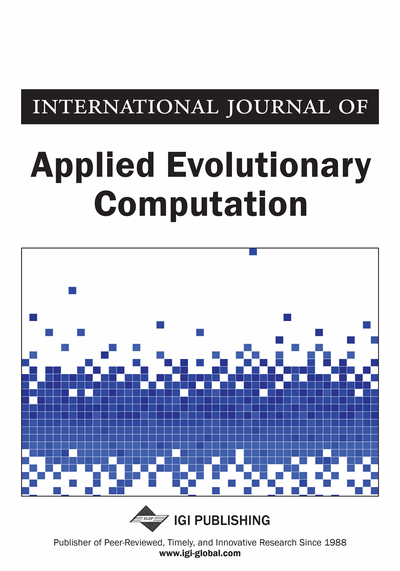# LZW Chromosome Encoding in Estimation of Distribution Algorithms

Orawan Watchanupaporn (Department of Computer Science, Kasetsart University, Sriracha Campus, Thailand) and Worasait Suwannik (Department of Computer Science, Kasetsart University, Bangkhen Campus, Thailand)
DOI: 10.4018/ijaec.2013100103

## Abstract

Estimation of distribution algorithm (EDA) can solve more complicated problems than its predecessor (Genetic Algorithm). EDA uses various methods to probabilistically model a group of highly fit individuals. Calculating the model in sophisticated EDA is very time consuming. To reduce the model building time, the authors propose compressed chromosome encoding. A chromosome is encoded using a format that can be decompressed by the Lempel-Ziv-Welch (LZW) algorithm. The authors combined LZW encoding with various EDAs and termed the class of algorithms Lempel-Ziv-Welch Estimation of Distribution Algorithms (LZWEDA). Experimental results show that LZWEDA significantly outperforms the original EDA. Finally, the authors analyze how LZW encoding transforms a fitness landscape.
Article Preview
Top

## Introduction

Estimation of distribution algorithm (EDA) is a new type of evolutionary algorithm (Mühlenbein & Paaß, 1996). EDA evolves a population in a more principled manner than its predecessor, genetic algorithm (GA) (Goldberg, 1989; Mitchell, 1996). In GA, randomness is used in many steps of the algorithm such as selecting highly fit individuals and creating a new population from selected individuals. GA creates a new individual by crossing over two selected individuals. The crossover point is chosen at random. After that, GA randomly mutates the new individual. However, in EDA, a new population is generated from a probabilistic model, which is built from highly fit chromosomes. Even though generating a new population in EDA also uses randomness, it is based on the knowledge about relationship among variables embedded in the model.

Building a model for a large problem (i.e., a problem with a solution encoded with a large number of bits) is very time consuming. For example, the running time for building a model for MIMIC and BOA is O(n2) and O(n2N+n3), where n is a chromosome length and N is the number of individuals used for building a model. Several approaches can reduce the size of the search space in evolutionary computation (Chen & Smith, 1999; Yong & Sannomiya, 2001). The authors have investigated compression as a search space reduction method for evolutionary computations. The authors’ earliest compressed encoding is similar to run-length encoding (Suwannik et al., 2005). The results demonstrate that the compressed GA requires 805 times less fitness evaluations than the simple GA when solving the 128-bit OneMax problem. Later, the authors proposed c2GA, which combines the compact genetic algorithm (cGA) with compressed encoding (Watchanupaporn et al., 2006). The performance of the c2GA is superior to that of cGA for the OneMax and RoyalRoad problems (Mitchell, 1994).

The compression algorithm used in compressed GA and c2GA is not effective. Moreover, the number of bits of the repetition times (the run length) must be specified. To overcome this problem, LZW-compressed chromosome encoding is proposed by Kunasol et al. (2006). An LZWGA chromosome is an array of integers that can be decompressed using the Lempel-Ziv-Welch (LZW) decompression algorithm (Welch, 1984). Because the size of compressed chromosome is typically smaller than the size of the decompressed chromosome, LZWGA searches a smaller search space. Consequently, it can be used to solve very large problems. Kunasol et al. (2006) conducted experiments on one-million-bit problems. Solving a problem of this size using any canonical GA is not practical. However, LZWGA could solve the one-million-bit OneMax and Trap problems in 160 generations. A one-million-bit problem has a search space size equal to 21000000, or 9.90x10301029, possibilities.

LZW compressed encoding was also applied to EDA. Watchanupaporn and Suwannik (2010) combines LZW compressed encoding with cGA, which is a univariate EDA. Unlike cGA, this algorithm does not use a probability vector to represent the entire population. Instead, it uses a probability matrix because the generated LZW chromosome is an array of integers. Watchanupaporn et al. (2012) proposed another method to combine LZW to cGA. Unlike the previous method, the core of the cGA algorithm does not have to be modified. Therefore, a probability vector can be used to represent the entire population. This method can easily be used in various EDAs such as LZWMIMIC (Watchanupaporn and Suwannik 2011) which combines LZW compressed encoding with MIMIC, which is a bivariate EDA (Bonet et al.,1997). This proposed algorithm outperforms the original MIMIC algorithm.

## Complete Article List

Search this Journal:
Reset
Open Access Articles: Forthcoming
Volume 12: 4 Issues (2021): Forthcoming, Available for Pre-Order
Volume 11: 4 Issues (2020): 3 Released, 1 Forthcoming
Volume 10: 4 Issues (2019)
Volume 9: 4 Issues (2018)
Volume 8: 4 Issues (2017)
Volume 7: 4 Issues (2016)
Volume 6: 4 Issues (2015)
Volume 5: 4 Issues (2014)
Volume 4: 4 Issues (2013)
Volume 3: 4 Issues (2012)
Volume 2: 4 Issues (2011)
Volume 1: 4 Issues (2010)
View Complete Journal Contents Listing